# How to choose glass rotor flowmeter

Glass Rotameter is a kind of instantaneous flow measuring instrument which is easy to use, easy to read and widely used. In the area of â€‹â€‹environmental protection equipment and equipment, the amount of up to 30,000 units/year. Therefore, it is extremely important to choose and use this type of instrument.

First, the glass to the flow meter of the variety and selection

The glass rotor flowmeter can be divided into: ordinary type, ribbed tube type, small flow rate and small size type, corrosion resistant type, laboratory type, heat preservation type, alarm type, and high pressure resistant type according to its use and adaptation range. series. According to the national series of instrument series, no matter which series, the maximum number includes 12 calibers from 1 mm to 100 mm. The measurable flow range is: liquid (water) 0.1 ml/min ~ 40 cubic meters/hour, gas (air) 1 ml/min to 1000 cubic meters/hour. The glass rotor flowmeter used for environmental protection equipment is generally not more than 10mm in diameter, and the measured flow is in a small flow range.

The selection of glass rotor flow meter can be considered from the following aspects.

1 The object of measurement. That is to measure the type of medium, pressure, chemical properties. Such as liquid medium, gas medium, corrosive media should be selected anti-corrosion flowmeter.
2 flowmeter itself performance. Generally speaking, after the above conditions are determined, if there is no major change in price, the needle valve may be preferentially placed on the upper part of the flow meter; if there is a large flow hole, it is a direct flow rate scale; the structure is simple; the external size is small, etc. . If it is a small flow range, ball floater type can be used, because it is stable when measuring, it is not easy to accumulate dust, has high precision and good interchangeability.
3 Select according to price. In general, the high accuracy and high price. According to the purpose of measurement, the instrument accuracy grade should be selected. For example, only the measurement medium throughput must be controlled. After trial operation adjustment, the throughput must be stabilized at a later time, then the accuracy is secondary.

Second, the calibration of the glass rotor meteor scale

The scale of the glass rotameter is obtained by the manufacturer using the near-ideal fluid water and dry air as the medium under the conditions of the factory. However, in the use of the flowmeter, there are two situations in which its scale value cannot be directly used: First, the measurement medium is not water and air, and second, the measurement medium is water and air, but its state (temperature, pressure) and scale state. Different. In this way, when using the flowmeter, in order to obtain correct measurement results, there arises the problem that the scale value needs to be corrected. Therefore, to solve the glass rotor flowmeter calibration correction is the key to using this instrument.

Considering that environmental instruments use rotameters to measure a large amount of gas flow, it is only discussed below for the density correction when measuring gaseous media. Since the viscosity of the gaseous medium is small, the effect of viscosity is omitted in the discussion. Practice has shown that this does not affect the accuracy of the correction.

The following is a general expression of the rotor flowmeter flow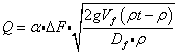In the formula, a - flow coefficient, dimensionless.
â–³F - The area of â€‹â€‹the annulus formed by the inner wall of the conical tube and the reading edge of the float changes with the height of the float (M2).
Vf - float volume (M3).
Df - The maximum cross section of the float (M2).
Î¡f - float density (kg/M3).
Ï â€”â€” Density of measured medium (kg/M3).
g - Gravity acceleration (9.81M/S2 - sea level).

Formula (1) is a formula that does not consider the influence of the viscosity of the medium. From equation (1), it can be clearly seen that when the height of a float of a flowmeter is determined, the density Ï of the measured medium is the only variable. If the density of the measured medium is different, the flow of the medium through the flowmeter is also different. Therefore, the scale correction is actually the flow rate correction.

If the two media with different densities Ï1 and Ï2 pass through the same rotor flowmeter, if the float is balanced on the same position, the rotor flowmeter density conversion basic formula of (1) is: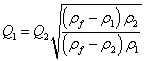(2)

For gases, Ïf>Ï1,Ï2,(2) can be simplified as: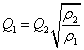(3)

Using the ideal gas law, (3) can be converted to: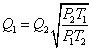(4)

In the formula, P1, T1 and P2, T2 are the two states of the same medium and are expressed by two parameters of pressure and temperature respectively. Visible, for the same kind of gas medium, the density conversion can be completely converted to different conditions of temperature, pressure conversion. In this way, the calibration of the measurement of the flow rate of the same gas medium eventually becomes the state correction of temperature and pressure (essentially the density correction). Obviously, this is an easy-to-implement correction method for general use units.

(a) How to determine the actual flow value from the reading of the flow meter scale at the site of use?

There is only one purpose of using a ready-made application to switch to the flowmeter: the actual flow of the measured medium. However, many users ignore the difference between the on-site status and the flowmeter calibration status (ie, the standard status), and directly use the flowmeter scale reading as the actual flow value of the measured medium. It is obvious that this actual flow is not true. It will cause errors in the measurement of the flowmeter, thus causing delays in the final test results of the supporting instruments.

In the field, the actual flow value is obtained from the flow meter scale reading, which is essentially the conversion of the flow rate value of the flow meter in the standard state to the flow rate in the field. We set parameters related to on-site operating conditions to actual flow Q, medium pressure P, and temperature T. The relevant parameter codes for the flowmeter scale of the rotor flowmeter are pressure P0 and temperature T0, respectively, compared with the standard state, according to (4):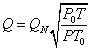Using formula (5), it can be seen that it is very convenient to obtain the actual flow value of the measured medium from the flowmeter reading at the scene. It needs to be pointed out that when calculating with formula (5), P, P0, T, and T0 should all be substituted into absolute values, and P is the pressure at the front of the meter, which should be measured on the upstream side of the flowmeter and immediately adjacent to the flow path of the flowmeter. .

Example: When using an air sampler, the rotameter on the sampler reads 500 ml/min, and the pressure before measuring the gauge is -100 mmH20 (using a suction pump, so it is a negative pressure). The on-site temperature is 30Â°C. Find the actual air flow value at this time.

Solution, based on the measured data,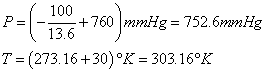then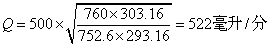Here, because of P. With millimeters of mercury as the unit, the millimeter water column must be converted into millimeters of mercury. For the calculation, only millimeters of water can be divided by 13.6.

From the above results, it can be seen that the difference between the on-site status and the standard status is not significant, but the measurement result yields a difference of 22 ml/min (4.4% of the standard value). In other words, if it is not corrected, the flow value will produce an error of 4.4%!

(b) How to choose the right rotameter for the user according to the actual use of the flow?

This problem is precisely the reverse of (a) above. It is only necessary to turn (5) into the reverse: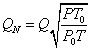Single Roller Corn Thresher

Single Roller Corn Thresher,Hand Crank Corn Sheller,Antique Corn Sheller,Electric Corn Sheller

Hunan Xinta Machinery Manufacturing Co., Ltd. , https://www.china-agricultural-product.com